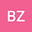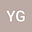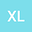An Effective Global Computational Algorithm for a class of Generalized Linear Multiplicative Programs
•••• +1
• Bo Zhang,
• Yuelin Gao,
• Xia Liu,
• Xiaoli Huang
Bo Zhang
Ningxia University

Corresponding Author:zbsdx121@163.com

Author ProfileYuelin Gao
North Minzu University
Author ProfileXia Liu
Ningxia University
Author ProfileXiaoli Huang
North Minzu University
Author Profile## Abstract

This paper explains a region-division-linearization algorithm for solving a class of generalized linear multiplicative programs (GLMP) with exponent. In this algorithm, the original non-convex problem (GLMP) is transformed into a series of linear programming problems by dividing the outer space of the problem (GLMP) into finite polynomial rectangles. A new two-stage acceleration technique is put in place to improve the computational efficiency of the algorithm, which removes part of the region of the optimal solution without problems (GLMP) in outer space. In addition, the global convergence of the algorithm is discussed, and the computational complexity of the algorithm is investigated. It demonstrates that the algorithm is a completely polynomial time approximation scheme. Finally, the numerical results show that the algorithm is effective and feasible.